The Multivariate EWMA Model and Health Care Monitoring | OMICS International
International Journal of Economics & Management Sciences

# The Multivariate EWMA Model and Health Care Monitoring

Xia Pan1 and Jeffrey E Jarrett2*

1Lingnan College, Sun Yat-sen University, Guangzhou, China

2Management Science (CBA), University of Rhode Island, Kingston, USA

*Corresponding Author:
Jeffrey E. Jarrett, Ph.D
Management Science (CBA)
University of Rhode Island
Kingston, RI 02881, USA
Tel: 401.874.4169;
E-mail: [email protected]

Received Date: April 29, 2014; Accepted Date: August 18, 2014; Published Date: August 28, 2014

Citation: Pan X, Jarrett JE (2014) The Multivariate EWMA Model and Health Care Monitoring. Int J Econ Manag Sci 3:176. doi:10.4172/2162-6359.1000176

Copyright: © 2013 2014 Pan X, et al. This is an open-access article distributed under the terms of the Creative Commons Attribution License, which permits unrestricted use, distribution, and reproduction in any medium, provided the original author and source are credited.

Visit for more related articles at International Journal of Economics & Management Sciences

#### Abstract

We introduce the construction of MEWMA (Multivariate exponentially weighted moving average) process control in the field of bio surveillance. Such introduction will both improve the reliability of data collected in bio surveillance, better interpretation of the results, improvement in the quality of results and standardization of results when more than two variables are involved. We propose sensitivity ratios as a measure of the effects of the mean shift and dispersion shift in processes under study. Using these sensitivity measures, we designed the optimal exponential weighting factor, which is consistent to results reported in control chart applications. Although ARL (average run length) is the usual measure for control chart performance in multivariate process control, it is by no means the only criterion, however, at the moment it is most widely used criterion for decision making. We suggest addition study of other criteria. For example Medial Run Length, Days to Completion, Direction of Eorrors and others.

#### Keywords

Multivariate EWMA; Sensitivity analysis; Serial correlation; Bio surveillance

#### Monitoring Bio Surveillance with MPC (Multivariate Process Control) Methodology

Modern bio surveillance involves the monitoring of a large number and wide range of data from samples of diagnostic and pre-diagnostic data. The purpose is to enable health care professionals to better recognize, detect, investigate and respond to the outbreaks of disease and epidemics. A central tool in this monitoring process in classical disease surveillance migrated to bio surveillance. The migration is the result of implementing multivariate quality control methods in the analysis. Fricker  applied multivariate statistical control methods with an application of MQC to syndromic surveillance. Fricker  continued the earlier study by focusing on directionally sensitive procedure in bio surveillance. Joner et al.  produced a one sided MEWMA (multivariate exponentially weighted moving-average) control chart for the analysis of health data. Yahav and Schmueli  introduced in practice directionally-sensitive MPC charts to bio surveillance methods. They examined four such techniques and came to conclusions based on simulated data, but suggested further research in the application of these methods.

Since bio surveillance is the process of monitoring health data concerning infectious diseases, the use of quality control and improvement methods largely developed in industrial application applies to the analysis of data collected in health care monitoring. Our purpose hence is to apply the useful methods to this type of control of infectious diseases in the very difficult procedures of preventing epidemics and other problems associated with the lack of adequate and ineffective safety programs in health care institution and public health programs. Traditional bio surveillance can take weeks, sometimes months, before detect ion and prevention measures are applied. We focus on one very useful method for such implementation of better methods.

Usually but not necessarily the data resulting from bio surveillance are counts and not measurements. The chief problem is often that broad categories are used such as gastrointestinal, unspecified infection, respiratory problems and/or neurological problems. These broad categories are often auto correlated with seasonal components, trends related to population changes and similar problems associate with other quantifiable components. Current analysis by Shewhart control charts which focus on a time series of a single variable whereby the result is either to conclude that the process is in control or not are often inadequate to reflect real change in the process results leading to misleading conclusions made by physicians and health care practitioners. We suggest a more modern approach that considers many of the problems not heretofore fully examined by contemporary quality control methods as part of a total quality management program. In the next section, we examine a methodology whereby better and more sophisticated results are possible.

#### Under MEWMA Modeling and Quality Control

Previously Ord et al.  recognized the usefulness of monitoring social or economic processes is a clear application of the notion of statistical process control (SPC). They extended the notions of control by Shewhart Control Charting to that of monitoring univariate time series. Furthermore, they suggested the use of EWMA charts for residuals, which will be effective in detecting level shifts and suggest their use in detecting shifts in variability. This improved process could also be explored by expanding the analysis to the multivariate case. Also they suggested that we examine the ideas explored by Lowry et al. , Pan and Jarrett  and Rungar et al. .

The multivariate form of the EWMA control chart simultaneously monitors two or more related process characteristics in an exponentially weighted control chart. For example, one uses a MEWMA chart to monitor temperature and pressure in a plastic injection-molding process. Each MEWMA point incorporates information from all the previous subgroups or observations in combination with a user-defined weighting factor. MEWMA charts can help you detect small process shifts quicker than other multivariate charts, such as the T-Squared control chart .

Another advantage of MEWMA charts is that they are not greatly influenced when a small or large value enters the calculation. Also, MEWMA charts can be custom tailored to detect any size shift in the process. In turn, difficult to detect small shifts, not detected by less sophisticated methods, one employs MEWMA control charts to find these small changes in a process.

Multivariate process control simultaneously monitors several processes in combination. A long list of manuscripts from 1995 until near the present consider the standard methods of Hoteling T-squared charts in various applications. In general, they studied industrial and commercial applications, however, the statistical properties of the data utilized in the studies are similar to those in health applications. A listing of the studies is given in a large number of papers in the quality monitoring literature [10-20], and finally Jarrett and Pan [21,22]. These monitoring methods employ the Hotelling T2 statistic for a current sample.

Exponentially weighted moving average (EWMA) charts which are more sensitive to moderate shifts in parameters than univariate charts are widely used in univariate cases [23,24]. LWCR extended the univariate EWMA control chart to the multivariate case by simulation. They noted that the multivariate EWMA (hereafter MEWMA) chart has greater sensitivity to shifts in the mean than more traditional Hotelling T2 control methods.

An alternative MEWMA scheme is Pan , which builds the Hotelling T2 of the variables before the formation of the EWMA of the T2 s. Lui  presented an improvement for MEWMA. Runger and Prahu  used Markov chain analysis to calculate the ARL for MEWMA and Prabhu and Runger  discussed the design of the same scheme. However, all these studies assumed the processes to be serially independent.

Others chose to study the usefulness of MEWMA methods as well. Stoumbus and Sullivan  investigated the effects of non-normality on the performance of the MEWMA control chart, and its special case, the Hotelling’s Chi-Squared control chart when applied to individual observations. The purpose in this case was to monitor the mean vector of a multivariate process variable. Khoo studied the sensitivity of MEWMA control charts under other circumstances. In addition, Scientists explored a method for optimally designing multivariate EWMA charts based on the measures of average run length (ARL) and median run length (MRL). In this study , we utilize the concept of sensitivity ratios based on the works of Otto et al.  and Väisänen and Hyttinen  who argued that sensitivity ratios are a superior method to assess quality in the areas of bioelectric measurements, plant disease-screening methods and others involving new technology. The sensitivity ratio is a statistic specifically developed for comparing for different measuring methods and is not based on any particular assumption about haw the measuring methods or scales are related. Hence, our purpose is to share new research in the evolution of monitoring processes by comparing results of experiments.

The Pan MEWMA scheme builds the Hotelling T2 of the variables before order of construction steps is the statistic of MEWMA chart. Pan  used integral equation method to compute the ARL’s of MEWMA charts for in-control and out-of-control situations without the presence of serial correlation. All MEWMA method variations are multivariate EWMA schemes.

The above schemes have a common problem, that is, they cannot be directly employed when the processes are serially correlated. An indirect way to apply the MEWMA schemes for serially correlated processes is to adopt Alwan and Roberts’  approach. They suggest estimating the residuals, i.e., one-step-ahead forecasting errors, of the autocorrelated process. In turn, they apply traditional control charts for the residuals. Extending this approach to multivariate cases, one can apply the above MEWMA scheme to the residuals of the serially correlated multivariate processes. The final result will have properly modeled processes, the initial number of observations will be sufficiently large and the residuals are asymptotically independent over time. Then, the EWMA At this point, we determine the sensitivity of these approaches to changes in process parameters in the presence of serial correlation. Since the process parameters are usually unknown, the appropriate estimation and use of the covariance matrix is vital for correct execution of MEWMA. This may occur if the direct sample variance is a biased.

We will in the next section, consider applications of MEWMA charts to monitor bio surveillance data to understand the meaning and application of these charts in a simulated experiment.

#### Under an Illustration of the MEWMA Control Chart

We consider a bio surveillance procedure where data is collected on five variables (A, B, C, D and E). The sensitivity of the process will be seen by the five control charts based on the dampening coefficient being equal to 0.1 to 0.6 and increasing and the level of 0.1. Recall that the exponential coefficient in MEWMA refers to relationship between the prediction and observed values of variables. The larger the value of the coefficient indicates the greater the influence of the error in the previous prediction. If the coefficient was 1, the prediction would be the same as in the previous time period.

Figures 1(a) through 3(d) provide for the results of the MEWMA charts yield different upper control limits (UCL) for each of the control charts. For example Figure 1(b) where the coefficient is 0.1, one find a UCL of 14.54 and out of control points of 5 through 13, 24 through 32 and 52 through 55.

Figure 1a: The Sensitivity Factor Srk for Mean Shift, large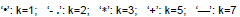Figure 1b: The Sensitivity FactorSrk for Mean Shift, large i (i=200).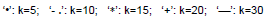Figure 1c: The Sensitivity Factor Srk for Mean Shift, small i (i=10).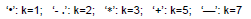Note when applying this method the value of the dampening coefficient is crucial.

For Figure 2, the UCL equals 15.73 but the number of points out of control becomes smaller. Only points6,8 9 and 23 through 30 are out of control. Figure 3(a) the UCL increases to 16.22and points 8 and 23 through 29 are out of control. For Figure 3(b) (coefficient of 0.4), the UCL equals 16.46 and points 8, and 24 through 27 are out of control. Figure 3(c) (coefficient equals 0.5, the UCL increases again to 16.60 and the points out of control 25 and 26. Finally, Figure 3(d) (coefficient equals 0.6) the upper bound is now the zero line and everything is out of control.

Figure 2: The Sensitivity η2 of M-EWMA Chart for Dispersion Shift, (i=40).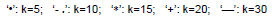Figure 3a: Srk (θ ) for Sensitivity η2 of M-EWMA Chart for Dispersion Shift, k=2 Staring State (i=10) .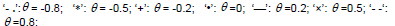Figure 3b: Srk (θ ) for Sensitivity η2of M-EWMA Chart for Dispersion Shift, k=5 Staring State (i=10)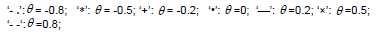Figure 3c: Srk (θ )for Sensitivity η2 of M-EWMA Chart for Dispersion Shift, k=10 Steady State (i=100).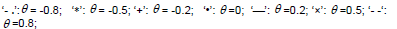Figure 3d: Srk (θ ) for Sensitivity η2 of M-EWMA Chart for Dispersion Shift, k=30 Steady State (i=100).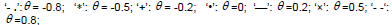#### MEWMA for Serially Independent Processes

For a p-dimensional multivariate an independent and identically distributed process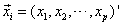at time point i, constructing the exponentially weighted moving-average (EWMA) quantities based on previous observations, we have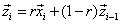(1)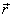is a 1 x i row vector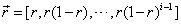Without losing generality, the mean in-control vector of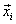is set at zero. We then construct the quadratic Hotelling T2 of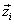as the chart statistic: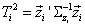(2)

where the covariance used in the T2 is the covariance of,and r was chosen as a scalar weighting parameter. It seems obvious that under the assumption of i.i.d. normal distribution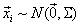with known parameters, the MEWMA or statisticfollows chisquare distribution with order of p, because eachis still normal. However, omitted here is that initial value of the EWMA,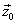.Ashas to be set as a certain value (say, 0), this may make the T21 a little different from the exact χ2(p).

To investigate the details of the distribution, we denote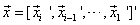be an ip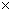1 column vector,be a 1i row vectorand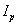are a pp unity matrix. For independent observations, we have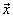~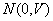with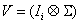Setting the initial EWMA vector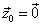we have,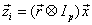(3)

and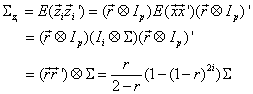(4)

where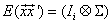because of the independence for observations at different time points Ii is a ii unity matrix), and that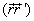is scalar. The result in (4) is the same as given in LWCR. Letting Rr be a normalized ii matrix that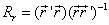the MEWMA or chart statistic is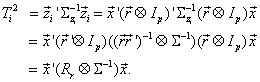(5)

We derive the distribution of (5) by applying the theorems on quadratic form discussed in Box  and others. Thus, the MEWMA in (5) is approximately distributed as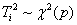and the distribution of (5) is irrelevant to the exponential weighting factor r. This result is consistent with the reported simulation results in LWCR. Therefore, the in-control average run lengths (ARL’s) and the control limits are the same as the results of LWCR for different values of r.

In addition, LWCR noted that the EWMA weighting parameter is a diagonal matrix with different elements, although they only reported the simulation results for scalar r. If the weights are different for different variables, the EWMA weighting parameter is a matrix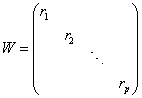(6)

We define a new ppi matrix, R=[W,W(Ip-W),Λ,W(Ip-W)i-1], to replace the previous Kronecker product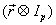in (3), so that (3) becomes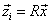Then, from the diagonal property of matrix RR’, it is not difficult to see that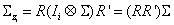(7)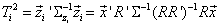(8)

If the r1,r2Λ,rp in W is the same r, (7) and (8) reduce to (4) and (5) respectively. In addition, (8) follows χ2(p), a chi-square determined by the number of dimensions of the system, and irrelevant to the EWMA weighting parameters for the variables. Since the scalar weight is just a special case of the matrix weight, (3) is a special case of (7).

#### Shift Effects and the Measure of Sensitivity - Mean Shift

If a mean shift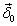occurs at i0=i-k, 0 ≤k ≤i, then we write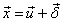, where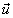is denoted as the in-control processes and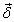is a Pi1 column vector that has δ0 in the first kp elements and zeros in the last i0p elements. Taking the scalar r case as example (the case of (6) can be discussed in the same way), the statistic of (5) becomes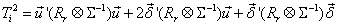(9)

This is a no central chi-square distribution. The first item in (9) is the in-control chi-square (note the mean vector of in-control processesis assumed to be zeros without losing generality). The second item is a normal distribution with mean of zero and variance of 4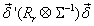.The second and the third items are all determined by the value of the third item which is a constant scalar. Defining the third item as Δ,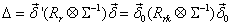(10)

Where Rrk, the upper-left kk partition matrix is Rr, partitioned according to shift occurrence time, i0. Obviously, (10) is relevant to r through Rrk. Therefore, the choice of the value of the exponential weight r in M-EWMA or does matter for the out-of-control distributions of the chart statistic.

By reexamining (10), it is actually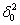times the sum of all the elements of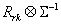Hence, it is not difficult to show that (10) is also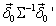times the sum of all the elements of Rrk . Denoting both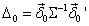and the sum of all the elements of Rrkas Srk we have Δ= Srk Δ0Note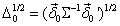is only the noncentrality parameter in LWCR. Δ0(or 1/2 Δ0 ) measures the size of the shift in the process itself instead of incorporating the control chart, while the measure Δ is chart-specific. It can be shown that the Srk is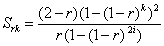(11)

Since the mean of (5) is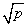and the mean of (9) can be viewed as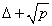the difference between them, Δ, can be viewed as the measure for the difference in distribution. Comparing this difference with the in-control dispersion measured by the in-control standard deviation,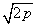we can design the sensitivity ratio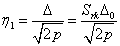(12)

to measure the performance of the control chart for detecting mean shift. As Srk is the only factor depending on r, we choose r for the optimal sensitivity through Srk. Figure 1(c) shows how the Srk changes with r for different occurrence time of the shift. For k=1, indicating a recent shift, exponential weighting will lower the sensitivity. Later, when k=2, weighting at r=0.5 gives the best sensitivity. For k is 3, r should be chosen at about 0.3. For k is around 5, r should be 0.2. For k is about 10, r should be 0.1. For k is as large as 30, r should be as small as 0.04. Our results indicate but do not resolve whether larger r is mandatory if the number of time intervals is small. Hence, these results are not fully consistent with the results based on ARL criteria in LCWR and Prabhu and Runger .

#### Shift Effects and the Measure of Sensitivity - Dispersion Shift

A shift in the dispersion parameter is a change in the process covariance matrix. Yeh, Huwang, and Wu studied a likelihood-ratiobased EWMA approach for multivariate variability. We now consider the MEWMA or chart by building the Hotelling T2 on the in-control covariance matrix. For out-of-control processes, the theoretical distribution which is the basis for constructing the chart is not clear. Moreover, if the mean ofis still zero, the theorems on quadratic form (see Appendix 1) are directly applicable. Suppose a shift from Σ to Σ1 occurs at i0=i-k, 0 ≤k ≤i, Since the covariance matrix is positively definite, there exists a matrix C so that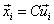and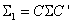after the time point i0. The chart statistic is still expressed in the form of (4) and (5) or (7) and (8) exceptis actually shifted. Since the covariance ofis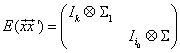(13)

The matrix U is (for common weight r, i.e. (4) and (5))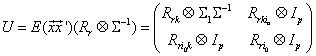(14)

Where Rrk, Rri0, Rrkiand Rri0k are partition matrices of Rr which is partitioned according to shift occurrence time i0. The eigenvalues of U depends on Rrk, Rri0 , and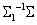and the eigenvalues of UU depends on all the four partitioned matrices of Rr. We can easily verify through simulation that the eigenvalues of the partitioned Rr does differ for varying values of r. Therefore, the distribution of the chart statistic is still chi-square but with parameter shift that depend on the exponential weight r (i.e., g and ν will be dependent on r instead of being 1 and p). The departure from the in-control statistic’s distribution determines how quickly on average the control chart detects the shift.

To design a sensitivity measure for the detection of the out-ofcontrol status due to dispersion change, we suggest the use of the ratio between the variance for the out-of-control and the variance for the in-control chart statistics. Since the in-control chart statistic is χ2(p) its variance is 2p. The variance of the out-of-control chart statistic is 2 trace (UU), where U is given in (14). We have the sensitivity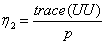(15)

Alternatively, we use the ratio of the standard deviations, which is the square root of (15), to measure sensitivity.

To show for purposes of comparison the effectiveness of r on sensitivity η2. Although may be used in practice, we illustrate by considering some particular cases that the 3-dimensional processes’ covariance matrix shifts from Σ to Σ1 at time point i0=i-k, 0 ≤k ≤i,.One of the cases is the dispersion shift is in such a way that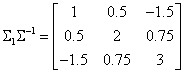(16)

Substituting (16) in (14), we calculate η2 for different r and k (k defined previously relating to the time point.). The result, shown in Figure 2, indicates that larger r yields higher sensitivity η2. If r is equal to unity (which be rare, if at all) it would not indicate that EWMA charts are not superior to ordinary Shewhart control charts [34,35]. We also examined several cases of Σ 1Σ−1. As long as the variance for each process increases (i.e. the diagonal elements of Σ 1Σ−1are great than one), larger r is preferred. This indicates that the current sample information is more important.

From the analysis, we see the selection of the exponential coefficient is crucial in the decision to conclude that the process is out of control or not. The process being the surveillance indicates that for MEWMA control charts the coefficient is a crucial parameter for assessing whether that each sample observation is in-control or out of control. Some solutions to this is to determine the optimal coefficient in the MEWMA and find that value for the coefficient that minimizes the sum of squares of the error term for the predations. This would be a multivariate least squares solution.

The 3-dimensional processes’ covariance matrix shifts from Σ to Σ1 at time point i0=i-k, 0 ≤k ≤i. One of the cases is the dispersion shift is in such a way that(16)

Substituting (16) in (14), we calculate η2 for different r and k (k defined previously relating to the time point.). The result, shown in Figure 2, indicates that larger r yields higher sensitivity η2. If r is equal to unity (which be rare, if at all) it would not indicate that EWMA charts are not superior to ordinary Shewhart control charts. We also examined several cases of Σ 1Σ−1. As long as the variance for each process increases (i.e. the diagonal elements of Σ 1Σ−1 are great than one), larger r is preferred. This indicates that the current sample information is more important. If at least one of the process variances decreases rather than increases, smaller r is preferred.

#### Under Summary

We studied the construction of MEWMA process control as it applies in health care applications.. We proposed sensitivity ratios as a measure of the effects of the mean shift and dispersion shift. Using this sensitivity measure, we designed the optimal exponential weighting factor, which is consistent to results reported before in control chart applications. Although ARL is the usual measure for SPC chart performance, it is by no means the only criterion, and it has shortcomings. Our proposed sensitivity measure has certain advantages. It is directly derived from the distributions of the process statistic; hence, it is not constrained. This makes the sensitivity measure have a broad range to fit varying situations. Furthermore, we see and examine the benefits of monitoring by MEWMA methods over the simpler univariate and EWMA methods.

We discussed the MEWMA chart on processes in presence of serial correlation by considering an adjustment to the best exponential weighting factor according to the information on serial correlation. In a future study, one may determine if the mean shift and variability shift occur at the same time and determine the magnitude of these effects. We can surmise that the issue if greatest when one effect is much greater than the other. However, this will be for future study. Future research, will be in the development of improvements in charts based on ARL. In depth knowledge on the distribution of the chart statistic may suggest a special way of constructing the variance-covariance matrix for the MEWMA scheme on multivariate moving-average processes. Using the sensitivity measure, we may investigate the role of serial correlation of the process in the structure of the chart statistic, and its impact on the sensitivity performance for a special process pattern (VMA (1)). This allows us to consider adjusting the optimal exponential weighting factor according to the information on serial correlation.

Future studies could examine the process monitoring when mean and variation shifts occur simultaneously. Which shift would have the greater effect on the monitoring the forecast and which shift dominates and at what levels? There is much to learn from using MEWMA especially in the light of economic and social processes which may have much great numbers of variables to consider than many applications in health sciences.

#### References

Select your language of interest to view the total content in your interested language

### Article Usage

• Total views: 12679
• [From(publication date):
September-2014 - Dec 08, 2019]
• Breakdown by view type
• HTML page views : 8808Can't read the image? click here to refresh